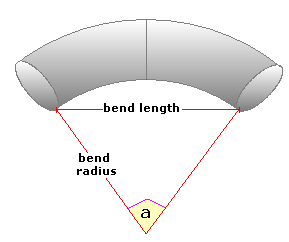Spike's Calculators

# Internal Angle from the Bend RadiusCalculate the internal angle of a curved section of pipe using the bend radius and the length of the curve. This calculator uses inches for measurements.

### Bend Angle - Bend Radius

Curve Length in
Decimal Precision #

#### Results:

 Angle in Radians rad Decimal Degrees ° Degrees Minutes Seconds ° ' "

#### Calculator

1. enter the length of the curve in inches
2. the bend radius in inches
3. decimal precision, the number of digits after the decimal point

#### Results

1. the internal angle in radians
2. in decimal degrees
3. in degrees, minutes, and seconds

##### Formula
```a = sin-1(d/r)
where a is the angle in radians
d half the length of the curve